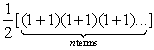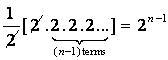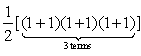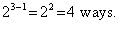1. Home
2. |
3. MBA |

# CAT Quantitative Ability – Composite Numbers

Nov 16, 2018 15:36 IST

CAT Quantitative Ability has questions on Composite Number. We know that every natural number greater than 1 can be written as a product of primes. The factorization is unique upto the order in which the primes are written. This means that the list of primes that occur in the factorization is unique (and the number of times each prime factor occurs in the list is unique). Only the order in which the primes are written can change.

Examples: 120 = 2 × 3 × 2 × 5 × 2
= 3 × 5 × 2 × 2 × 2 = 2 × 2 × 2 × 5 × 3 etc.

57330 = 7 × 13 × 3 × 2 × 7 × 5 × 3

• If the distinct prime factors of a number m are p, q, ..., r and the number of times that they divide m are a, b, ..., c respectively, then we can write

m = pª qb … rc

Examples: 120 = 2⊃3; × 3 × 5

57330 = 2 × 32 × 5 × 7⊃2; × 13

• Now, if we wish to resolve m into two factors prime to each other, then ‘of the two factors’ one must contain pa  (otherwise there would be some power of p in one factor and some power of p in the other factor — and the two factors will not remain prime to each other).  Similarly, we need to understand that qb should occur in one of the factors of m only…and so on and so forth! What this implies is that — the required number, m is equal to the number of ways in which the product ‘pqr…’can be resolved into two factors.

The number of ways in which m can be resolved into two factors, therefore, iswhere n is the number of different prime factors

in m

Or• Example: In how many ways can the number 7056 be resolved into two factors prime to each other?

Finding the prime factors of 7056, we get

(i.e., there are 3 different prime factors: 2, 3 & 7)

The number of ways in which 7056 can be resolved into two factors, therefore, iswhere 3 is the number of different prime factors in

7056.

Or, The number of ways in which 7056 can be resolved into two factors isThis concept comes in handy while you are attempting numerical questions in quantitative ability section. This gets applied in many questions.

So this was all about the composite numbers exercise! If you want to read more about other concepts that are asked in the quantitative aptitute sections in MBA entrance exams such as CAT, XAT, CMAT, MAT and others, then stay tuned to MBA section of jagranjosh.com

CAT quantitative aptitude concepts: Percentages

## Register to get FREE updates

All Fields Mandatory
• (Ex:9123456789)
•